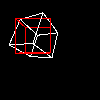Name

modelZ()

Examplessize(100, 100, P3D)
noFill()

background(0)
pushMatrix()
# start at the middle of the screen
translate(width/2, height/2, -100)
# some random rotation to make things interesting
rotateY(1.0)#yrot)
rotateZ(2.0)#zrot)
rotateX(0)
# offset from center
translate(0, 50, 0)
# draw a white box outline at (0, 0, 0)
stroke(255)
box(50)
# the box was drawn at (0, 0, 0), store that location
x = modelX(0, 0, 0)
y = modelY(0, 0, 0)
z = modelZ(0, 0, 0)
# clear out all the transformations
popMatrix()
# draw another box at the same (x, y, z) coordinate as the other
pushMatrix()
translate(x, y, z)
stroke(255, 0, 0)
box(50)
popMatrix()
Description Returns the three-dimensional X, Y, Z position in model space. This returns the Z value for a given coordinate based on the current set of transformations (scale, rotate, translate, etc.) The Z value can be used to place an object in space relative to the location of the original point once the transformations are no longer in use.

In the example, the modelX(), modelY(), and modelZ() functions record the location of a box in space after being placed using a series of translate and rotate commands. After popMatrix() is called, those transformations no longer apply, but the (x, y, z) coordinate returned by the model functions is used to place another box in the same location.
Syntax
modelZ(x, y, z)
Parameters
x float: 3D x-coordinate to be mapped float: 3D y-coordinate to be mapped float: 3D z-coordinate to be mapped
Related modelX()
modelY()

Updated on Mon Sep 21 15:53:25 2020.

If you see any errors or have comments, please let us know.